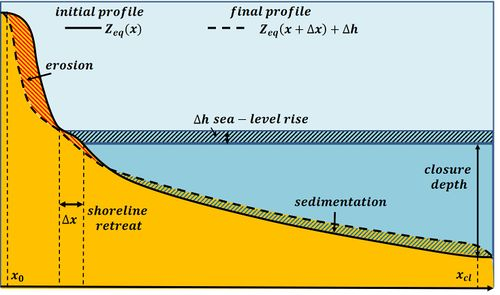# Bruun rule for shoreface adaptation to sea-level rise

(diff) ← Older revision | Latest revision (diff) | Newer revision → (diff)

The basic idea of Per Bruun when he proposed in 1954 the rule for shoreface adaptation to sea-level rise refers to the concept of equilibrium profile . The shoreface of a sedimentary coast should not be considered a geologically inherited feature, but the result of the natural coastal response to modern hydrodynamic conditions, as noted already more than a century ago by Cornaglia (1889)  and Fenneman (1902) . For a more detailed explanation, see the article Shoreface profile.Figure 1. Schematic representation of the shoreface shift in response to sea-level rise. The shaded eroded and deposited volumes are equal. The vertical/horizontal scale ratio is greatly exaggerated.

If climate change affects only the sea level, while leaving the wave climate unchanged, Bruun reasoned that the equilibrium shoreface profile slope $Z_{eq} (x)$ then should also remain the same. The profile should move upward, however, to keep the same position with respect to sea level as before. Lifting the profile requires supply of sand. Bruun assumed that sand supply from offshore is negligible if the shoreface profile extends seaward up to the closure depth. The sand should then be supplied by the landward part of the active coastal profile, i.e. the dry beach and the foredune. This implies shoreline retreat (designated $\Delta x$) and a corresponding landward shift of the equilibrium shoreface profile. The new equilibrium profile $Z^{new}_{eq} (x)$ is then equal to the old equilibrium profile $Z_{eq} (x)$ with an upward shift equal to the sea-level rise $\Delta h$ and a landward shift $\Delta x$ (see Fig. 1):

$Z^{new}_{eq} (x) = Z_{eq} (x+\Delta x) + \Delta h . \qquad(1)$

The shoreline retreat $\Delta x$ can be computed by the requirement that the total sand volume in the active zone (between $x_0$ and $x_{cl}$, see Fig. 1) remains the same:

$\int_{x_0}^{x_{cl}} [Z_{eq} (x+\Delta x) + \Delta h] dx = \int_{x_0}^{x_{cl}} Z_{eq} (x) dx . \qquad(2)$

Assuming that $\Delta x$ is small we have

$Z_{eq} (x+\Delta x) \approx Z_{eq} (x) + \Delta x \; \Large \frac{d Z_{eq} (x)}{dx} \normalsize . \qquad(3)$

Substitution in equation (2) then yields for the horizontal landward shoreline shift $\Delta x$ the Bruun rule

$\Delta x \approx \Delta h \; \Large \frac{x_{cl} – x_0}{Z_{eq} (x_0) – Z_{eq} (x_{cl})} \normalsize . \qquad(4)$

The Bruun rule has been widely applied, but the results did often not compare very well to observations. Critical comments have been written on the Bruun rule . A major reason for discrepancies results from ignoring the assumptions underlying the derivation of the Bruun rule. This holds in particular for the assumption that the sand volume in the active coastal profile remains unchanged: sand supply from other sources and sand losses are not considered. It is clear, however, that the Bruun rule can easily be extended to include other sand sources and sand losses .

We call $\Delta V$ the volume change [$m^3/m$] of the active coastal profile due to sand loss (positive) and sand supply (negative) during the time that the sea level rises by $\Delta h$. This quantity should be added in the left hand side of Eq. (2). We then find for the horizontal landward shoreline shift $\Delta x$:

$\Delta x \approx \Delta h \; \Large \frac{x_{cl} – x_0}{Z_{eq} (x_0) – Z_{eq} (x_{cl})} + \frac{\Delta V}{Z_{eq} (x_0) – Z_{eq} (x_{cl})} \normalsize . \qquad(5)$

Causes of sand loss and sand supply are:

• Sand loss to the dunes, by aeolian transport;
• Overwash of low coastal barriers;
• Transport across the closure depth contour;